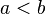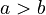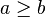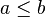# Inequality facts for kids

Kids Encyclopedia Facts

Inequality is when one object is:

• Smaller than the other ($a means that a is smaller than b)
• Bigger than the other ($a>b$ means that a is bigger than b)
• Not smaller than the other ($a\geq b$ means that a is not smaller than b, that is, it is either bigger, or equal to b)
• Not bigger than the other ($a\leq b$ means that a is not bigger than b, or it is smaller or equal to b)

Inequality is sometimes used to name a statement that one expression is smaller, greater, not smaller or not greater than the other.

## Working with inequalities

Inequality in math is when two solutions or answers are compared by greater than or less than. It is when the two or yet many solutions are being compared is not of equal amount. Solving an inequality means finding its solutions. When you substitute a number to a variable and the statement is true, then it is a solution. When you substitute a number to a variable and the statement is not true then, the number is not a solution to the statement.

Solving inequality is finding a solution to a given variable. It is finding a relative order of a set. Inequality might have many solutions, but usually only solutions as real numbers are the ones we are looking for. The proper way to read inequality is from left to right, just like the other equations, but the only difference is that they have different rules for every equation.

For example, consider the inequality x+4>12, where x is a real number. First, one needs to find the x and verify that it is actually a solution. The answer will be x>8 and it is a true statement. This expression is about the location of x within the set of real numbers. A number line is one way to show the location relative to all other real numbers.(See figure Inequality 1)

## Different kinds of inequalities

There are five different kinds of inequalities:

1. The first kind is linear inequalities, which are inequalities that differentiate the expressions by either less than or equal to, less than or greater than or equal to, greater than. It is one that if we replace the inequality for the equals relation, then the outcome will be a linear equation.
2. The second kind is the combinations of inequalities which are to satisfy the inequalities. Here, one must first have a number in the solution sets, so that the numbers satisfying the inequalities are going to be the values in the crossing of the two solution sets.
3. The third kind is inequalities involving absolute values, which means that the values can be rephrased as combinations of inequalities that will involve absolute values.
4. The fourth kind is called polynomial inequalities, which means that it is continuous and that their graphs do not have any jumps or breaks.
5. Last but not the least, is the rational inequalities, which means that it is the form of one of the polynomial divided by another polynomial. In other words, the rational function graphs do not have any breaks nor represent at the zeros of the denominator.

## Four ways to solve Inequalities

There are four ways to solve quadratic equations:

1. Add or subtract the same number on both sides.
2. Shift the sides and change the positioning of the sign of the inequality.
3. Multiply the same number on both sides.
4. Divide the same positive or negative number into the both sides.

Furthermore, it will take two steps to solve an inequality. The first one is to simplify using the reciprocal of addition or subtraction. The second one is to simplify more by using the reciprocal of multiplication or division. Note that when multiplying or dividing an inequality by a negative number, the inequality symbol must be reversed.

## Examples of how to solve Inequalities

Inequality is a mathematical statement that explains that the two values are not equal and are different. The equation ab means that a is not equal to b. Inequality is the same with any equation, with the (only) difference that inequality does not use an equal sign but inequality symbols. The inequality b>a means that b is greater than a. Speed limits, mark, and others use inequality to express them.

When solving an inequality, a person need to have a true statement. When you divide or multiply an inequality with a negative number on both sides, the statement is false. In order to make the statement correct with a negative number, one must reverse the direction of the inequality symbol. When a number is a positive number, one does not need to reverse the symbol. Inequality is about making a true statement.

For example, start with a true statement -6y<-12. When both sides are divided by -6, the result will become y<2. In this statement, the symbol need to be reversed in order to have a true statement, which produces y>2 as the correct answer. In the number line (see figure Inequality 2), a closed shaded circle points out that it is included in the solution set. An open circle points out that it is not included in the solution set.

## Related pagesInequality Facts for Kids. Kiddle Encyclopedia.